## Introduction to the UncertainInterval package

### Identifying test scores that are most uncertain

Medical tests (or bio-markers) are designed to distinguish patients with a targeted condition from the patients without that condition. For this classification, most often a dichotomous threshold is determined, allowing for two classes: patients with and without the targeted disease. This decision is often made without considering the possible uncertainty of the patient’s classification. Although it is common knowledge that the highest and the lowest scores leads to a decision with the least uncertainty and that scores somewhere in the middle lead to the most uncertain classification, this knowledge is seldom applied when determining cut-points.

The uncertain interval methods allow for the determination of an interval of test scores that are the most uncertain for distinguishing patients with and without the targeted condition. Typically, a patient with such a test score would either receive additional tests (when available) to obtain more certainty about the true status of the patient, or further developments of symptoms can be awaited. The latter approach can vary from regular active surveillance to informing the patient which changes in symptoms should trigger further diagnostics.

An uncertain test score has another weighing of costs than solely a decision for or against the presence of the disease. When the existence of the disease is uncertain, the benefits of treatment would be highly uncertain while the risks of possible negative side effects of a treatment remain present.

In this vignette, first the theoretical basis of the uncertain interval method is explained. Next, an example dataset is analyzed to illustrate the various possibilities of the method. The use of PSA scores as an early indicator of prostate cancer are discussed as an example.

NOTE 1: This example is intended to illustrate the determination of uncertain or inconclusive test scores. It is not intended to promote the use of PSA as a single indicator.

The PSA test is a relatively weak test, and demonstrates the clear existence of an interval of uncertain test scores. Prostate cancer in itself is often a slow growing form of cancer and patients with this disease do not necessarily die from the disease. On the other hand, prostate cancer treatments can have serious side effects that seriously affect the quality of life, such as incontinence and sexual dysfunctions, without necessarily leading to a longer life span. Therefore, the current policy is to prevent over-treatment and to offer patients active surveillance of their disease. The question which PSA scores should lead to informing the patient that prostate cancer is very unlikely, which test scores should lead to immediate further diagnostics and possible treatment and which test scores should lead to active surveillance is therefore relevant. The intention of this vignette is to illustrate how the functions in the UncertainInterval package can be used to answer this kind of questions.

### Intersection and Overlap

Tests are not perfect and uncertainty whether the test can distinguish the patients is dependent on the test score. Preferably, tests have a single point on intersection between the two distributions of patients with and without the targeted condition. In that case, high and low test scores are the most certain, but test scores in the middle are far less certain. The test scores at the point of the intersection of the two distributions is most uncertain (figure 1). The point of intersection is the point where the densities of the two distributions are equal. On the point of intersection, the probability that the patient belongs to the population of patients with the targeted disease (H1) is equal to the probability that the patient belongs to the population of patients without the disease (H0).

intersect.binormal <- function(m1, sd1, m2, sd2, p1=.5, p2=.5){

B <- (m1/sd1^2 - m2/sd2^2)
A <- 0.5*(1/sd2^2 - 1/sd1^2)
C <- 0.5*(m2^2/sd2^2 - m1^2/sd1^2) - log((sd1/sd2)*(p2/p1))

if (A!=0){
(-B + c(1,-1)*sqrt(B^2 - 4*A*C))/(2*A)
} else {-C/B}
}

x = seq(-5,8,length=1000)
mu0=0; sd0=1; mu1=2; sd1=2
y0 <- dnorm(x,mu0, sd0)
y1 <- dnorm(x, mu1, sd1)
is=intersect.binormal(mu0, sd0, mu1, sd1)
plot(x,y0,type='l', col='green', xlab='predictor', ylab='density', main='Figure 1')
lines(x,y1,type='l', col='black')
legend('topright', legend=c('H0', 'H1'), lty=c(1,1), col=c('green', 'black'), cex=.7)
threshold = qnorm(.90, mu0, sd0) # allowing a FPR of .1
abline(v=c(is, threshold), col=c( 'red', 'black'))
text(1.9, 0.03, expression(alpha), col='green')
text(0, 0.03, expression(beta), col='black')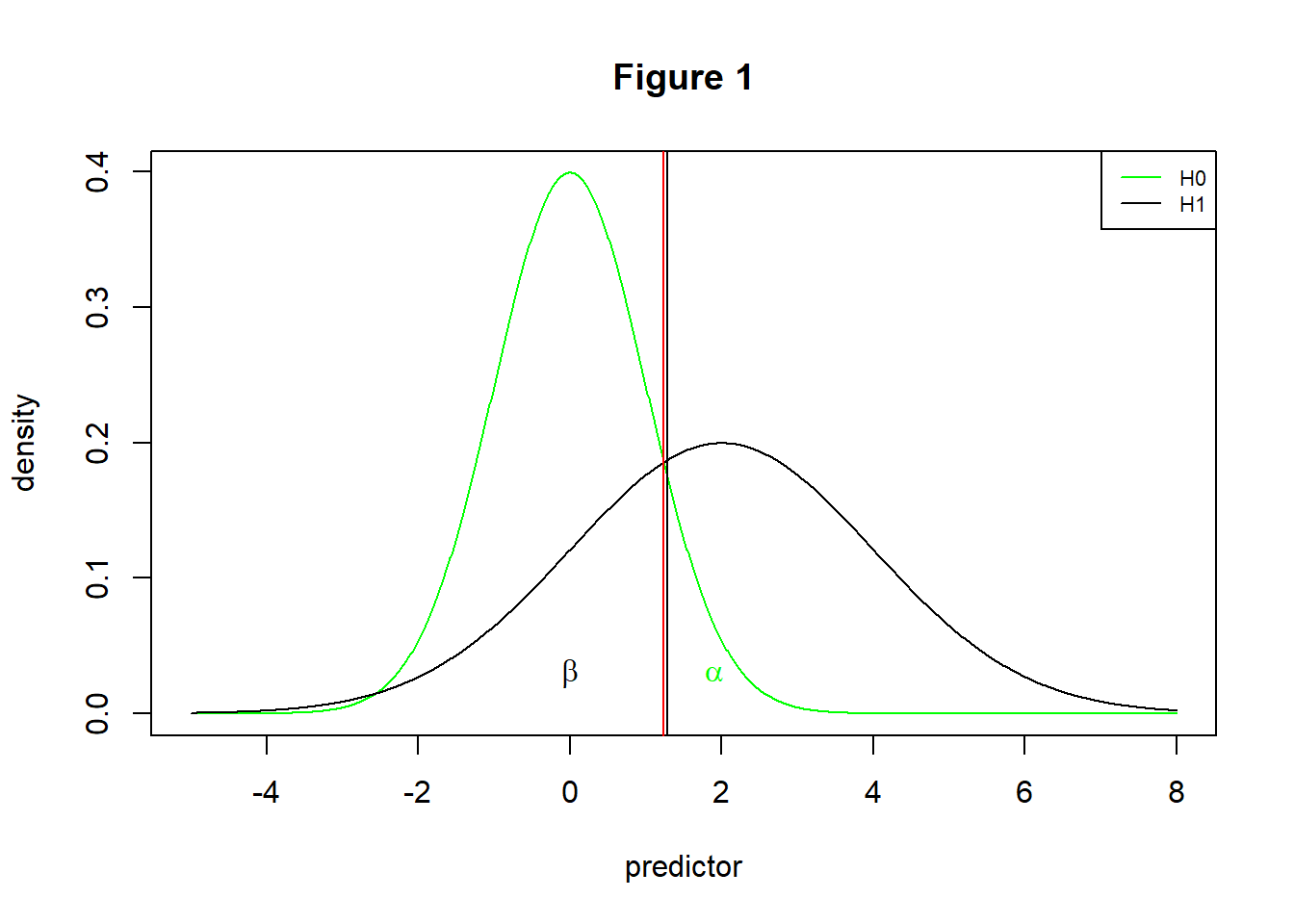Around the intersection, the test scores are found that are the most uncertain. Uncertain test scores are therefore defined as test scores where the densities of the two distributions are almost equal.

Let us define a threshold (black line) close to the intersection (red line). For the patients that have test scores close to the intersection, the probability to receive a wrong classification is near 50%. The classification based on a test score close to the intersection is least valid: a second measurement is bound to give results slightly lower or higher. When a patient has a score slightly above the intersection, when measuring again the result can be slightly below the intersection. The classification would reverse, while in reality little has changed.

The intersection is also the point where the sum of false positive rate (FPR = $$\alpha$$ (alpha error) = 1 - Sp) and false negative rate (FNR = $$\beta$$ (beta error) = 1 - Se) is minimal (Schisterman et al., 1995). The intersection is therefore the same as the Youden threshold, the point where the sum of true positive rate (TPR = Se) and true negative rate (TNR = Sp) is maximal. Small differences may occur as a result of differences in estimation methods, but in principle the intersection and the Youden threshold are the same.

NOTE 2: Tests that have more than one intersection can be problematic. When an intersection occurs in a region where the densities are very low, such an intersection can be ignored. When this is not the case, extra intersections indicate a lower quality of the test. An extra intersection indicates the existence of a region where the interpretation of results is reversed and not straightforward.

The uncertain interval methods offer the possibility to determine an interval that can be considered to be too uncertain to distinguish one type of patient from the other type. This indicates to the decision maker that more information is needed before a classification can be made. The decision maker is far less uncertain about a patient with scores outside the defined middle range of test scores (Landsheer, 2016, 2018).

### An Example

An example (Figure 2) is provided, using a file of the PSA bio-marker for the identification of prostate cancer (Etzioni R, Pepe M, Longton G, Hu C, Goodman G, 1999):

library(UncertainInterval)
data(psa2b)
names(psa2b)
#>  "id"   "d"    "t"    "fpsa" "tpsa" "age"
plotMD(psa2b$d, psa2b$tpsa)
abline(v=4, col='red')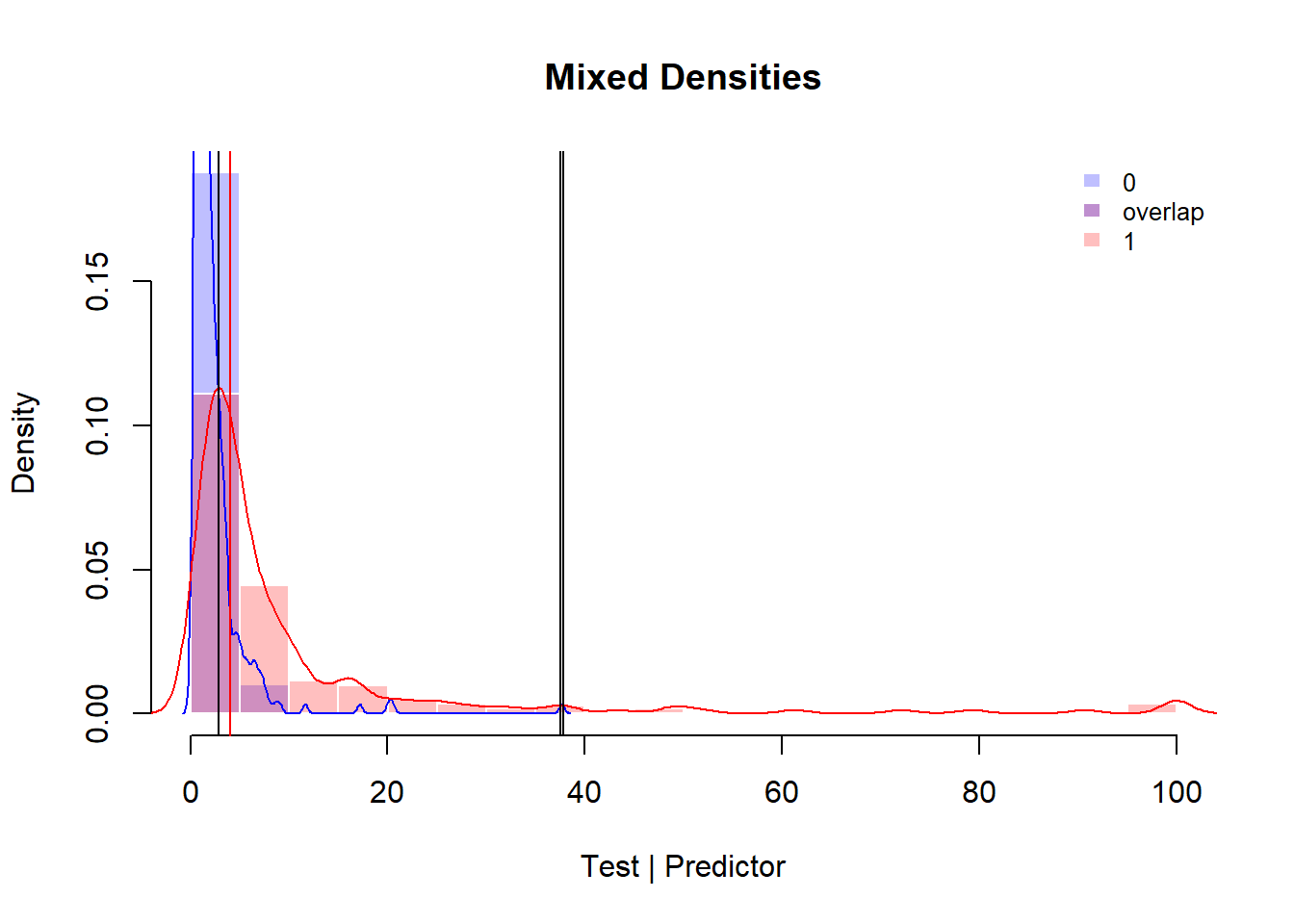Figure 2

It is easy to see in figure 2 that the interpretation of these distributions of scores is problematic. A large overlap is found in the lower region, both distributions have long tails and there are multiple intersections. Both patients with (1) and without (0) prostate cancer can have low or high PSA scores. Using the usual threshold of 4 micro gram per liter (red line), it is easy to see that there are a considerable number of miss classifications and especially the number of false negatives (FN = 101) is high when compared to the number of true positives (TP = 128), resulting in a low sensitivity of .56. Table 1 shows the confusion matrix of all 683 patients.

t2 = table (psa2b$tpsa > 4, psa2b$d)
rownames(t2) <- c('PSA <= 4', 'PSA > 4')
library(knitr)
kable(addmargins(t2), caption = "Table 1", padding = 20)
Table 1
0 1 Sum
PSA <= 4 414 101 515
PSA > 4 40 128 168
Sum 454 229 683

The higher the PSA score, the more certain the presence of prostate cancer is, but patients without prostate cancer can have high PSA values as well. Only the highest PSA values (> 40) leave little doubt that these patients have prostate cancer, but choosing such a high threshold makes that the percentage of false negatives increases greatly.

Determination of the most uncertain test scores may help. The function ui.nonpar dynamically calculates the sensitivity and specificity of the test scores around the intersection to determine the lower and upper threshold of this interval of uncertain scores. This function uses the rank order of the sampled test scores and is non-parametric. The default values for the sensitivity and specificity of the test scores within the uncertain interval is .55, which means that the ratios TP/FN and TN/FP are .55/(1-.45) = 1.22.

This interval is located around the intersection, which is determined in the ui.nonpar function by density estimation. The estimation of the point of intersection is therefore semi-parametric. If needed, this estimation can be overridden by setting the intersection parameter of the ui.nonpar function.

(res=ui.nonpar(psa2b$d, psa2b$tpsa))
#> Warning in ui.nonpar(psa2b$d, psa2b$tpsa): More than one point of intersection. Highest density used.
#>         cp.l         cp.h           FN           TP           TN           FP
#>    2.3600000    3.3000000   13.0000000   16.0000000   27.0000000   22.0000000
#>        UI.Se        UI.Sp intersection
#>    0.5517241    0.5510204    2.8375843

Please note that a warning is generated. A second point of intersection is found. We ignore these secondary points, as there are low densities there.

The thresholds found are 1.92 and 4.00 and 27 + 33 + 55 + 45 = 160 observations are considered as uncertain or indeterminate. The function quality.threshold provides the quality indices of the lower and upper less uncertain intervals (called MCI’s or More Certain Intervals). The MCI’s concern the patients who actually receive a classification for or against the possible presence of the targeted condition. Patients with an uncertain test score do not receive such a classification. A classification as uncertain points to the necessity of additional data before making a decision. In this case (using the default uncertain interval), the number of false negatives is reduced to 41 of the 355 patients who receive a classification against the targeted condition.

(out=quality.threshold(psa2b$d, psa2b$tpsa, res, res))
#> $direction #>  "<" #> #>$table
#>                             ref
#> class                          0   1 Sum
#>   0 (test < threshold.lower) 345  56 401
#>   Uncertain / NC              49  29  78
#>   1 (test > threshold.upper)  60 144 204
#>   Sum                        454 229 683
#>
#> $cut #> threshold.lower threshold.upper #> 2.36 3.30 #> #>$indices
#>  Prevalence     MCI.CCR MCI.balance      MCI.Sp      MCI.Se     MCI.NPV
#>   0.3352855   0.8082645   4.2155172   0.8518519   0.7200000   0.8603491
#>     MCI.PPV    MCI.SNPV    MCI.SPPV     MCI.LR-     MCI.LR+       MCI.C
#>   0.7058824   0.7565427   0.8263310   0.3218024   4.7580786   0.8543210

The sensitivity for the patients who receive a decision for or against the disease has increased to .76. It was 128/228 = .56, so there is a big improvement but it is still a sensitivity that is wanting. Specificity is reduced slightly to .89, as the uncertain interval deselects 55 (TN) + 45 (FP) = 100 non-patients, for which the test score is considered as too uncertain.

NOTE 3: In this case, the uncertain test scores are left out for the calculation of Se and Sp. The indicators Se and Sp are developed for use with a single cut-point. Using the upper and lower bound of the uncertain interval as single cut-points would lead to considering the uncertain test scores as classification errors, which would be nonsensical.

NOTE 4: The size of the uncertain interval is open for discussion. In the case of PSA it is certainly possible to argue for a wider interval of uncertain test scores.

The function quality.threshold.uncertain provides detailed quality indices for the uncertain interval when applied to the sample. These indices show a low quality, indicating that these test scores provide very uncertain information about the true status of these patients.

(t2 = quality.threshold.uncertain(psa2b$d, psa2b$tpsa, res, res))
#> $direction #>  "<" #> #>$intersection
#>  2.837584
#>
#> $table #> ref #> UI.class 0 1 Sum #> 0 (threshold.lower <= test < intersection) 27 13 40 #> 1 (intersection <= test <= threshold.upper) 22 16 38 #> Sum 49 29 78 #> #>$cut
#> threshold.lower threshold.upper
#>            2.36            3.30
#>
#> $indices #> Proportion.True UI.CCR UI.balance UI.Sp UI.Se #> 0.3717949 0.5512821 1.2285714 0.5510204 0.5517241 #> UI.NPV UI.PPV UI.SNPV UI.SPPV UI.LR- #> 0.6750000 0.4210526 0.5514085 0.5513361 0.8135377 #> UI.LR+ UI.C #> 1.2288401 0.5686137 It is easy to see in figure 3, that the scores of the patients in the uncertain interval have a relatively flat distribution and can hardly be distinguished from each other. Patients form both distributions have similar or slightly lower or higher scores. sel = psa2b$tpsa >= res & psa2b$tpsa <= res plotMD(psa2b$d[sel], psa2b$tpsa[sel])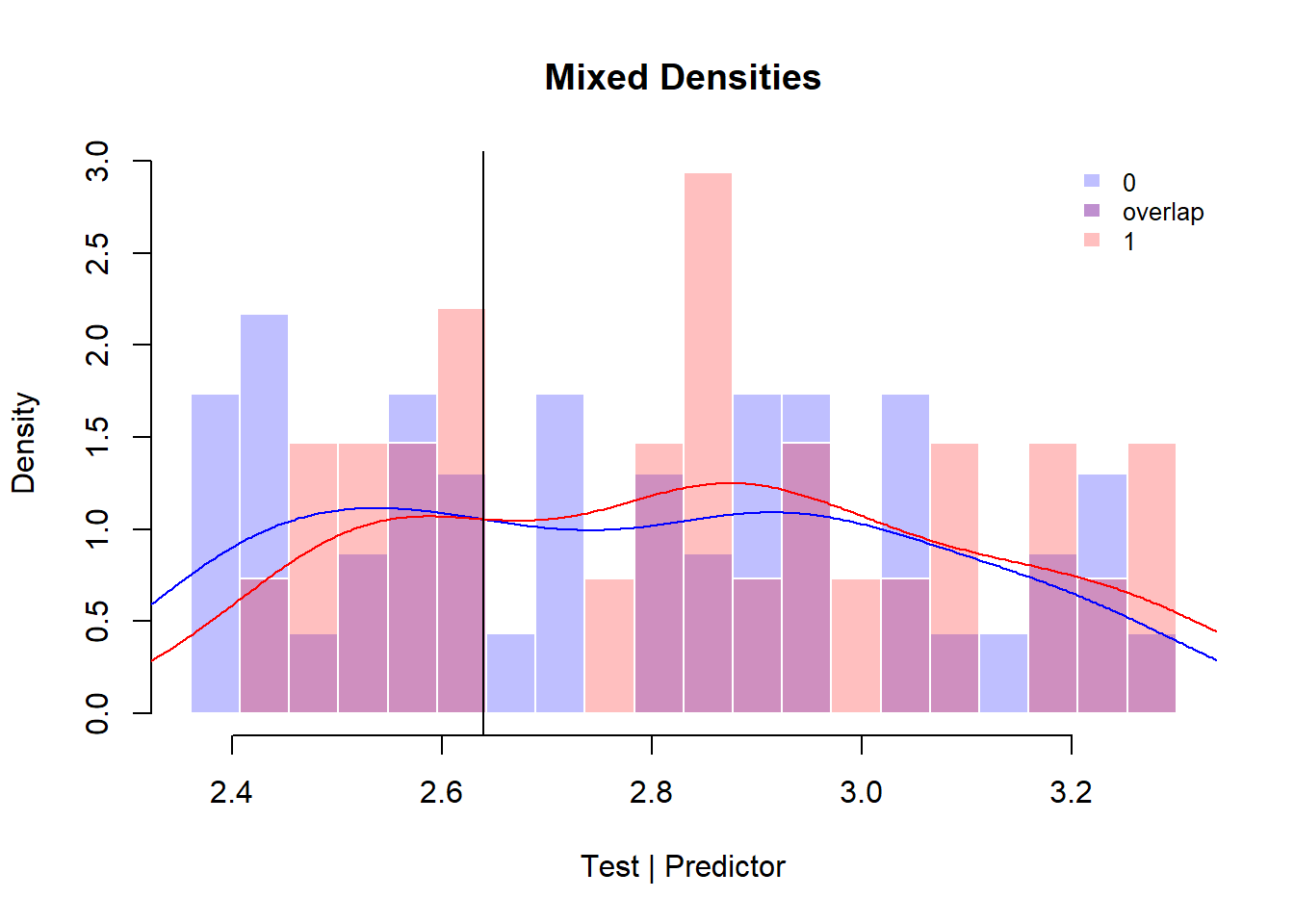Figure 3 Table 2 shows the confusion table of the scores within the interval (using the intersection as threshold): kable(t2$table, caption = "Table 2")
Table 2
0 1 Sum
0 (threshold.lower <= test < intersection) 27 13 40
1 (intersection <= test <= threshold.upper) 22 16 38
Sum 49 29 78

### How much uncertainty?

The question how much uncertainty is acceptable, depends on the clinical setting. The severity of the illness, the possibilities to treat, possible side effects of the treatments and the availability of better, perhaps more expensive bio-markers are relevant parameters. All these clinical parameters influence the decision how much uncertainty is acceptable and should lead to additional testing or active surveillance while waiting for possible further development of symptoms, respectively how much certainty is necessary to make a more definitive decision for or against the presence of the disease.

In the case of prostate cancer, the cancer often grows slowly and is often not causing any symptoms, while treatment with surgery or radiation has serious risks such as incontinence and impotence and does not necessarily prolongs life. Active surveillance with examinations each six months or awaiting possible changes in symptoms is often the more desirable line of action. In that case, we need a high certainty for decisive actions and might accept a wider range of test scores that we consider as diagnostically insufficient. As the PSA bio-marker is a relatively weak indicator, a wide range of test scores in the middle offers insufficient indication for treatment or no treatment. In general, a diagnostic insufficient indication can lead to the decision to use better or additional measurements that offer a better indication.

A wider uncertain interval is obtained by increasing the values of specificity and sensitivity of the uncertain test scores. Higher values make the test scores in the uncertain interval less uncertain, while the test scores outside the uncertain interval provide increased certainty, that is an increased percentage of correct decisions considering the true status of the patient. In the next code block, specificity and sensitivity is increased to respectively .60, .65 and .70.

(res=ui.nonpar(psa2b$d, psa2b$tpsa, UI.Se = .60, UI.Sp = .60))
#> Warning in ui.nonpar(psa2b$d, psa2b$tpsa, UI.Se = 0.6, UI.Sp = 0.6): More than one point of intersection. Highest density used.
#>         cp.l         cp.h           FN           TP           TN           FP
#>     2.790000     2.860000     2.000000     3.000000     3.000000     2.000000
#>        UI.Se        UI.Sp intersection
#>     0.600000     0.600000     2.837584
quality.threshold(psa2b$d, psa2b$tpsa, res, res)$indices[c('MCI.Sp', 'MCI.Se')] #> MCI.Sp MCI.Se #> 0.8218263 0.7008929 (res=ui.nonpar(psa2b$d, psa2b$tpsa, UI.Se =.65, UI.Sp = .65)) #> Warning in ui.nonpar(psa2b$d, psa2b$tpsa, UI.Se = 0.65, UI.Sp = 0.65): More than one point of intersection. Highest density used. #> cp.l cp.h FN TP TN FP #> 1.3700000 7.2800000 47.0000000 87.0000000 134.0000000 72.0000000 #> UI.Se UI.Sp intersection #> 0.6492537 0.6504854 2.8375843 quality.threshold(psa2b$d, psa2b$tpsa, res, res)$indices[c('MCI.Sp', 'MCI.Se')]
#>    MCI.Sp    MCI.Se
#> 0.9596774 0.7684211

(res=ui.nonpar(psa2b$d, psa2b$tpsa, UI.Se =.70, UI.Sp = .70))
#> Warning in ui.nonpar(psa2b$d, psa2b$tpsa, UI.Se = 0.7, UI.Sp = 0.7): More than one point of intersection. Highest density used.
#>         cp.l         cp.h           FN           TP           TN           FP
#>    1.1600000   19.3500000   57.0000000  133.0000000  184.0000000   79.0000000
#>        UI.Se        UI.Sp intersection
#>    0.7000000    0.6996198    2.8375843
quality.threshold(psa2b$d, psa2b$tpsa, res, res)$indices[c('MCI.Sp', 'MCI.Se')] #> MCI.Sp MCI.Se #> 0.9842932 0.6923077 The function quality.threshold produces several statistics to describe the qualities of the test scores outside the uncertain interval (MCI or More Certain Intervals). In this case, when increasing the uncertain interval, outside the uncertain interval the specificity improves faster than the sensitivity. However, in the last case, the sensitivity deteriorates for the more certain interval (MCI) outside the defined uncertain interval. The reason for this deterioration is that the number of correctly identified true patients does hardly increase, while the total number of true prostate cancer patients with scores outside the uncertain interval becomes smaller. Increasing the uncertain interval should therefore not be applied blindly. ### Usage tip: using negated values The UncertainInterval package uses an uniform definition of thresholds. When a single threshold is applied, test scores >= threshold indicate patients with the targeted disease and test scores < threshold indicate patients without the targeted disease. When an uncertain interval is determined, test scores >= lower threshold AND <= upper threshold are considered as uncertain. Test scores < lower threshold indicate the absence of the targeted disease and test scores > upper threshold indicate the presence of the targeted disease. NOTE 5: In the UncertainInterval package it is assumed that the test values of the patients with the targeted condition are larger than the test values of the patients without the targeted condition. When this is not the case, when lower values indicate the presence of the targeted condition, one can simply negate the test values by putting a minus sign before the test values. This makes the interpretation straightforward; all quality indices remain applicable. If one wants to report the non-negated test values (for instance when plotting the distributions), it is sufficient to discard the negative sign. Of course, relative comparisons, such as test score -3 > -5, reverses: 3 < 5. When a single threshold of -4 is determined, test scores >= -4 indicate patients with the targeted disease. When one wants to report the non-negated value, test scores <= 4 indicate patients with the targeted disease. ### Using transformations When using a diagnostic test, the ultimate goal is to apply the test to new patients, other than the patients used for verification of the method. Therefore, generalization is as important for any test as is its sensitivity, specificity or other validation indicators. Most often, a parametric model is optimized for the samples used but lacks a population model. Consequently, a non-parametric approach of a test necessitates considerable larger samples for validation to ensure that the quality indicators of the test results are not overly adapted to a small sample with particular characteristics. Generalization is easier when a valid parametric model can be applied, such as the bi-normal model. The advantage of a valid parametric model for the test is that we have a population model that allows for greater precision, accuracy and power. Results of a representative sample can be used to estimate population values and can be more easily generalized to other representative samples. The best results for generalization are achieved when a test is developed from the ground up with the use of a specific parametric model and a well defined population in mind. Unfortunately, this is not so often the case for medical tests. In figure 2 it is easy to see that the PSA test is not very good in identifying true patients but also not good in identifying patients without prostate cancer. Another approach of this data-set is the use of a transformation, so that a parametric method can be used. From a statistical point of view, we can ask whether a transformation of these test scores could be used to improve on the distribution. In this case, we can use the Box-Cox transformation (available in the car package) to improve the distributions (figures 4 & 5). library(UncertainInterval) if (!require(car)) install.packages("car",dependencies=TRUE) #> Loading required package: car #> Loading required package: carData library(car) data(psa2b) p1 = powerTransform(psa2b$tpsa)
t_tpsa = bcPower(psa2b$tpsa, p1$roundlam)

qqPlot(t_tpsa[psa2b$d==0]) #>  116 362 qqPlot(t_tpsa[psa2b$d==1])
#>  200 214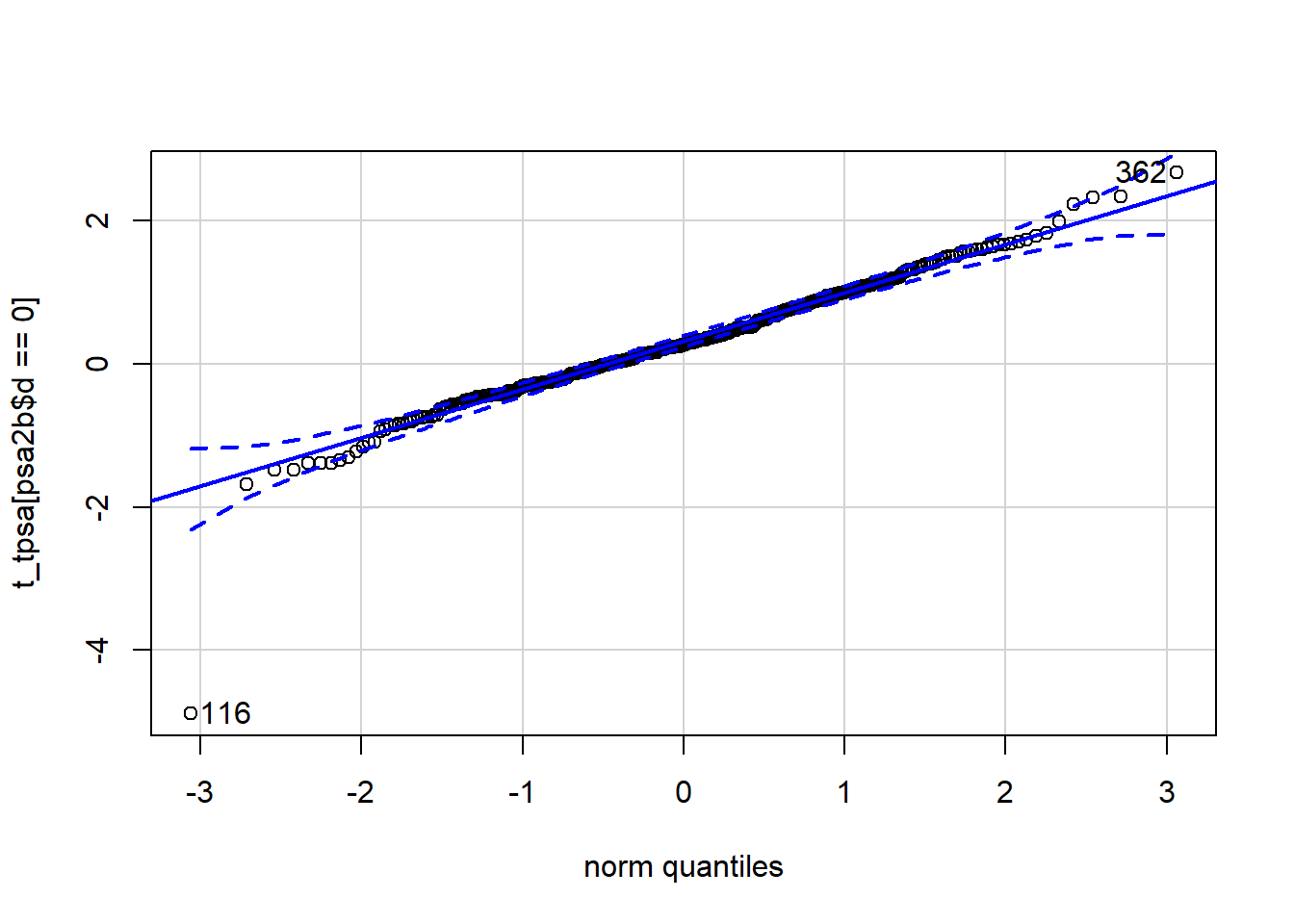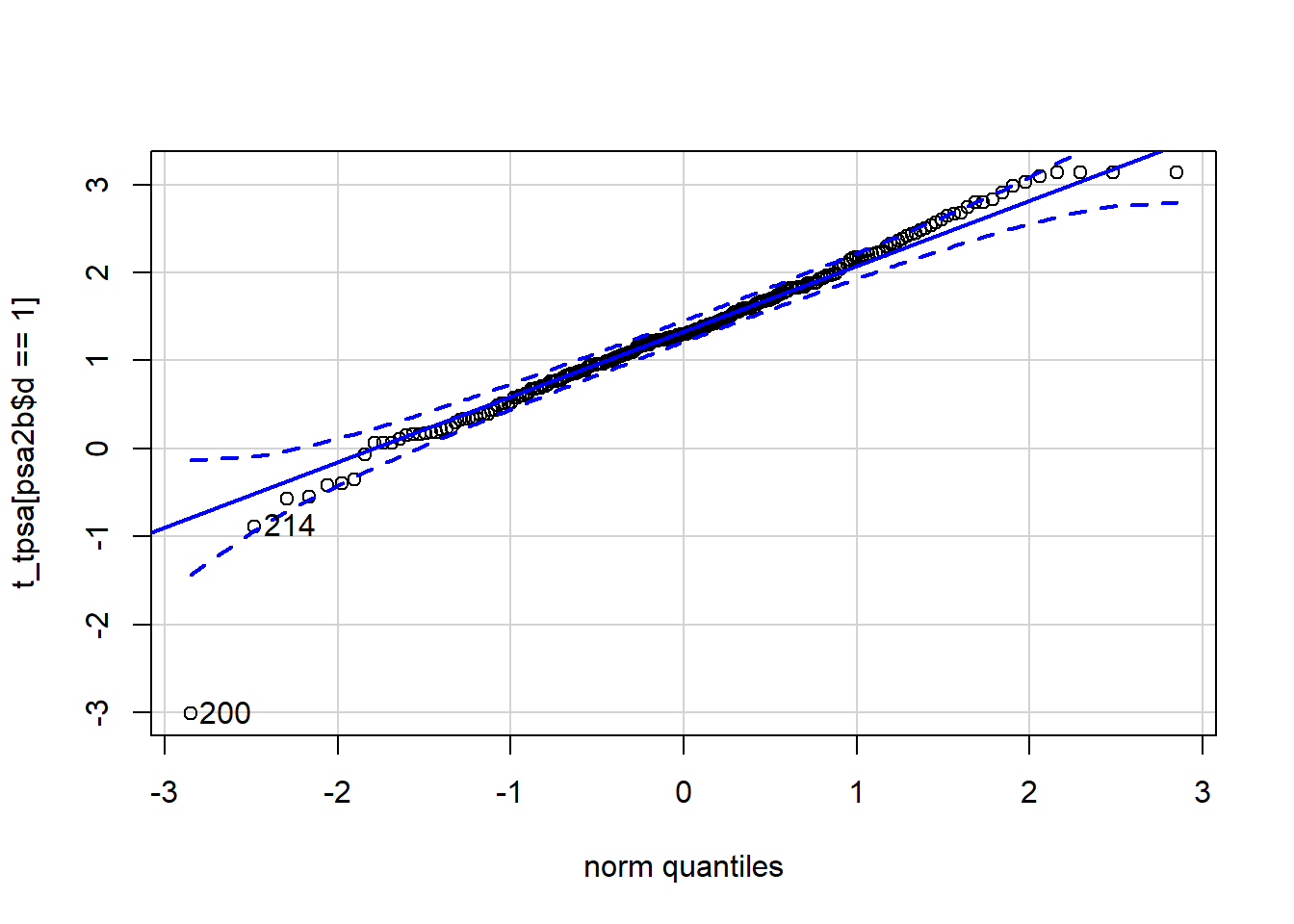Figures 4 & 5

The Box-Cox transformation limits skewness of the data by applying a power transformation. This transformation maintains the rank order of the data, but changes its distribution. The method searches for the power transformation that results in the lowest variance. The intention of the method is to transform the data to a shape that is more similar to a normal distribution. There is no guarantee, but the two qq plots show that a reasonable approximation of normal distributions is reached.

Figure 6 shows how the data are approximated using a bi-normal model.


plotMD(psa2b$d, t_tpsa, model='binormal', position.legend = 'topleft') (res1=ui.binormal(psa2b$d, t_tpsa))
#> Warning in nlopt.ui(UI.Se = UI.Se, UI.Sp = UI.Sp, mu0 = m0, sd0 = sd0, mu1 =
#> m1, : More than one point of intersection. Point with highest density used.
#> $status #>  4 #> #>$message
#>  "NLOPT_XTOL_REACHED: Optimization stopped because xtol_rel or xtol_abs (above) was reached."
#>
#> $intersection #>  0.8597443 #> #>$results
#> exp.UI.Sp exp.UI.Se       mu0       sd0       mu1       sd1
#> 0.5500000 0.5500000 0.3002297 0.7219207 1.3208021 0.8378606
#>
#> $solution #> L U #> 0.6318927 1.0991342 abline(v=res1$solution, col= 'red')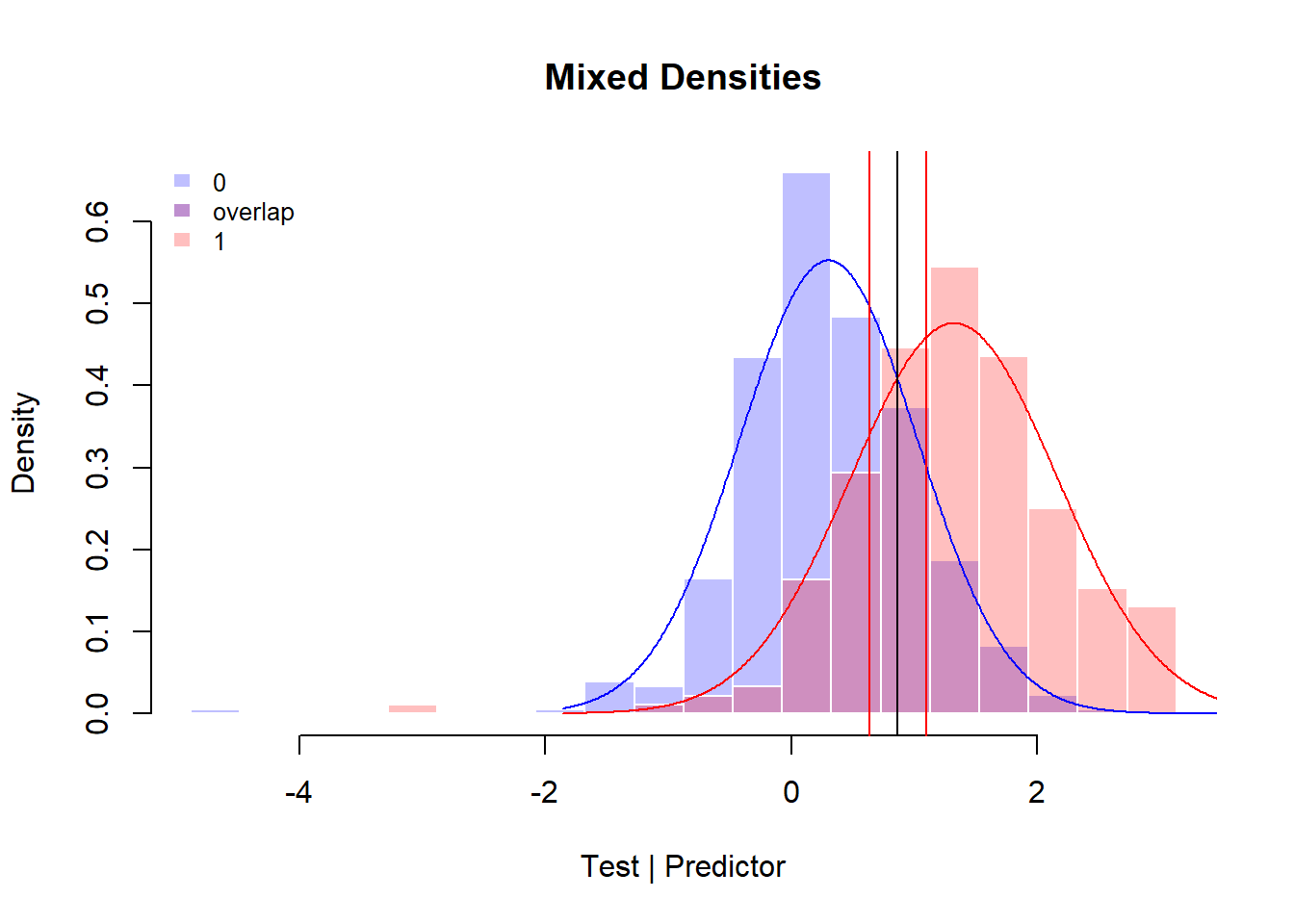Figure 6


invBoxCox <- function(x, lambda)
if (lambda == 0) exp(x) else (lambda*x + 1)^(1/lambda)
invBoxCox(res1$solution, p1$roundlam)
#>        L        U
#> 1.955678 3.402414

Of course, not all problems have disappeared; outliers are still there. The boxplot in figure 7 shows that the outliers in the lower tail are problematic: low scores < -3 indicate both a patient and a non-patient. These extreme scores in the sample also influence the estimates of the bi-normal distributions somewhat.

outlier_values <- boxplot.stats(t_tpsa)$out # outlier values. inds <- which(t_tpsa %in% outlier_values) table(outlier_values, psa2b$d[inds])
#>
#> outlier_values      0 1
#>   -4.88500502959075 1 0
#>   -3.01290516092602 0 1
#>   2.98492123652568  0 1
#>   3.02834906992807  0 1
#>   3.08915246880567  0 1
#>   3.13226431569987  0 1
#>   3.13230804246717  0 3
boxplot(t_tpsa, main="", boxwex=0.1)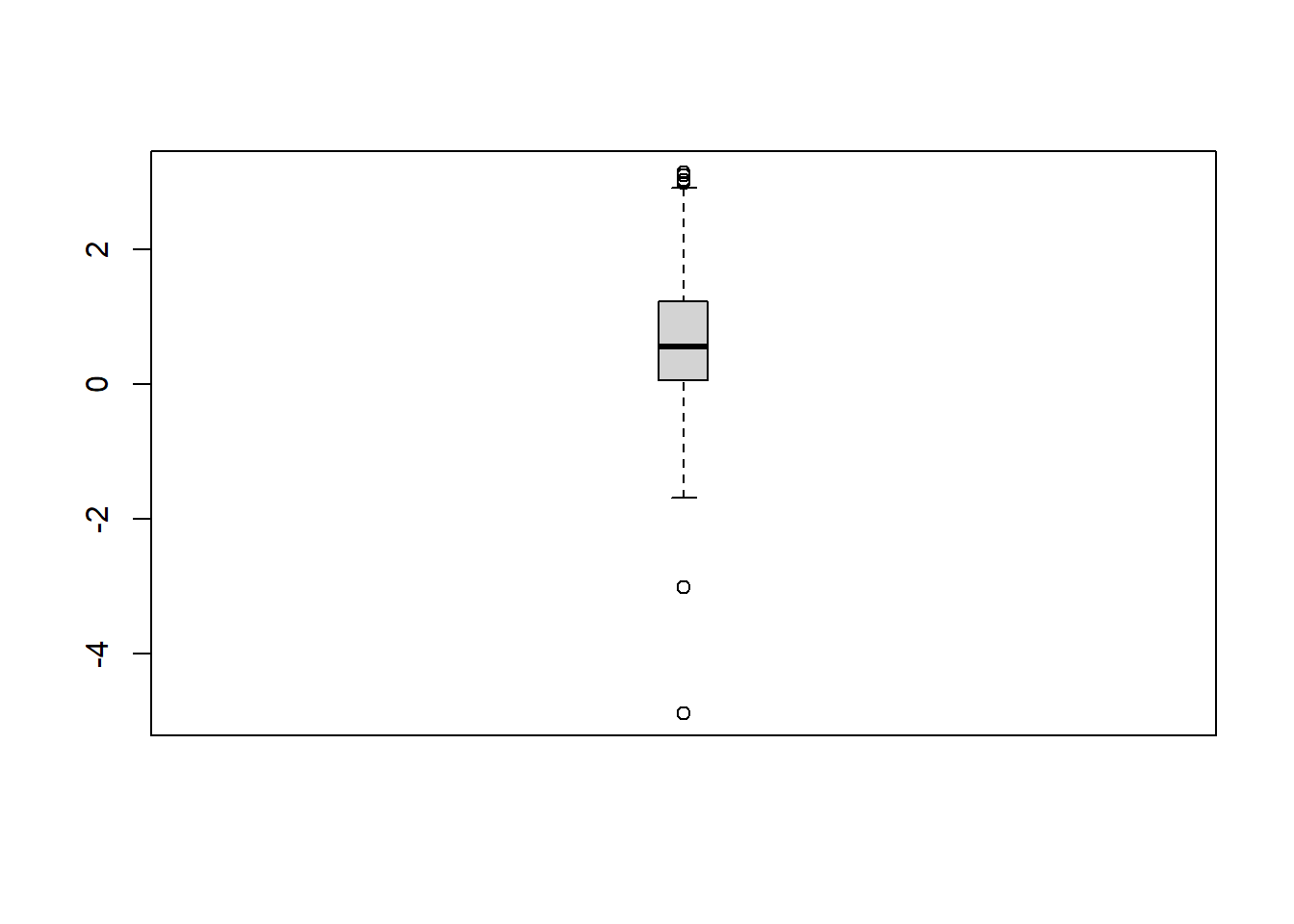Figure 7

# mtext(paste("Outliers: ", paste(round(outlier_values, 2), collapse=", ")), # cex=0.6)

Leaving out these extreme values may improve the estimates.

NOTE 6: the exercises here are intended as illustrations how the UncertainInterval package can be used in the case of a test with a difficult distribution. The PSA test is not developed with this transformation or the bi-normal model in mind. Whether this transformation is the best way to deal specifically with PSA test scores would require additional research to ascertain that the transformation has more general validity. Furthermore, there is considerable discussion about the usefulness of PSA scores for early identification of prostate cancer. Some researchers have argued that the PSA test may have better predictive value for specific age sub-populations (Sadi, 2017).

sel=t_tpsa > -3
plotMD(psa2b$d[sel], t_tpsa[sel], model='binormal') (res55=ui.binormal(psa2b$d[sel], t_tpsa[sel]))
#> Warning in nlopt.ui(UI.Se = UI.Se, UI.Sp = UI.Sp, mu0 = m0, sd0 = sd0, mu1 =
#> m1, : More than one point of intersection. Point with highest density used.
#> $status #>  4 #> #>$message
#>  "NLOPT_XTOL_REACHED: Optimization stopped because xtol_rel or xtol_abs (above) was reached."
#>
#> $intersection #>  0.8641356 #> #>$results
#> exp.UI.Sp exp.UI.Se       mu0       sd0       mu1       sd1
#> 0.5500000 0.5500000 0.3116761 0.6802260 1.3398096 0.7886725
#>
#> $solution #> L U #> 0.6625423 1.0749633 abline(v=res55$solution, col= 'red')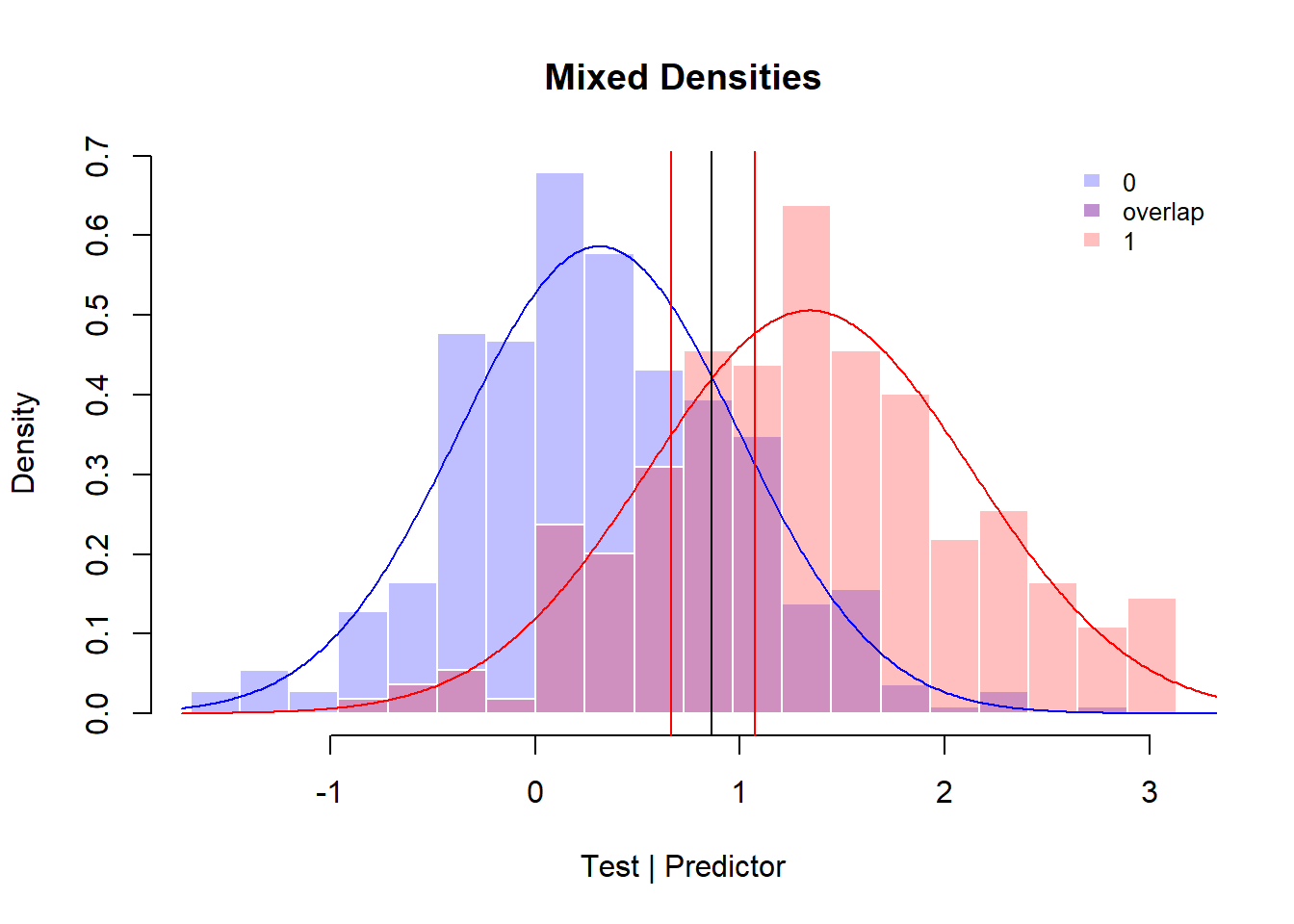invBoxCox <- function(x, lambda)
if (lambda == 0) exp(x) else (lambda*x + 1)^(1/lambda)
invBoxCox(res55$solution, p1$roundlam)
#>        L        U
#> 2.024697 3.301731

Of course, a wider range of test scores can be considered as diagnostically uncertain. In the next block we compare higher values for sensitivity and specificity of the uncertain test scores and show the obtained results for the outer, more certain regions:

res60=ui.binormal(psa2b$d[sel], t_tpsa[sel], UI.Se=.60, UI.Sp=.60) #> Warning in nlopt.ui(UI.Se = UI.Se, UI.Sp = UI.Sp, mu0 = m0, sd0 = sd0, mu1 = #> m1, : More than one point of intersection. Point with highest density used. res60$results
#> exp.UI.Sp exp.UI.Se       mu0       sd0       mu1       sd1
#> 0.6000000 0.6000000 0.3116761 0.6802260 1.3398096 0.7886725
quality.threshold.uncertain(psa2b$d, t_tpsa, res60$solution, res60$solution)$indices[c('UI.Se',  'UI.Sp')]
#>     UI.Se     UI.Sp
#> 0.7142857 0.4927536
quality.threshold(psa2b$d, t_tpsa, res60$solution, res60$solution)$indices[c('MCI.Se',  'MCI.Sp')]
#>    MCI.Se    MCI.Sp
#> 0.7793103 0.8860759

res65=ui.binormal(psa2b$d[sel], t_tpsa[sel], UI.Se=.65, UI.Sp=.65) #> Warning in nlopt.ui(UI.Se = UI.Se, UI.Sp = UI.Sp, mu0 = m0, sd0 = sd0, mu1 = #> m1, : More than one point of intersection. Point with highest density used. res65$results
#> exp.UI.Sp exp.UI.Se       mu0       sd0       mu1       sd1
#> 0.6500000 0.6500000 0.3116761 0.6802260 1.3398096 0.7886725
quality.threshold.uncertain(psa2b$d, t_tpsa, res65$solution, res65$solution)$indices[c('UI.Se',  'UI.Sp')]
#>     UI.Se     UI.Sp
#> 0.7307692 0.5779817
quality.threshold(psa2b$d, t_tpsa, res65$solution, res65$solution)$indices[c('MCI.Se',  'MCI.Sp')]
#>    MCI.Se    MCI.Sp
#> 0.7878788 0.9406780

res70=ui.binormal(psa2b$d[sel], t_tpsa[sel], UI.Se=.70, UI.Sp=.70) #> Warning in nlopt.ui(UI.Se = UI.Se, UI.Sp = UI.Sp, mu0 = m0, sd0 = sd0, mu1 = #> m1, : More than one point of intersection. Point with highest density used. res70$results
#> exp.UI.Sp exp.UI.Se       mu0       sd0       mu1       sd1
#> 0.7000000 0.7000000 0.3116761 0.6802260 1.3398096 0.7886725
quality.threshold.uncertain(psa2b$d, t_tpsa, res70$solution, res70$solution)$indices[c('UI.Se',  'UI.Sp')]
#>     UI.Se     UI.Sp
#> 0.7303371 0.6655738
quality.threshold(psa2b$d, t_tpsa, res70$solution, res70$solution)$indices[c('MCI.Se',  'MCI.Sp')]
#>    MCI.Se    MCI.Sp
#> 0.8431373 0.9731544

The statistics estimated for the uncertain interval of the modeled distribution are exactly what we asked for: sensitivity and specificity are respectively .60, .65 and .70. These estimates are no longer solely based on the sample and the values in the sample deviate, as can be expected. In the last example, with sensitivity and specificity set to .7, the sample values are respectively .73 and .67. When the bi-normal model is valid for these test scores, the bi-normal estimates of .7 can be considered as better, but this requires additional prove.

The obtained sample values for the outer regions show systematic improvements when sensitivity and specificity for the uncertain test scores are higher. These sample values are provided by the quality.threshold function. In the last case, specificity has increased to .97 while sensitivity has increased to .84.

A further question is how many patients are considered as having an uncertain test result, when using these values for sensitivity and specificity for determination of the uncertain interval. The extended confusion tables are produced by the quality.threshold function. The diagnoses based on the uncertain test scores are labeled as NA:

quality.threshold(psa2b$d, t_tpsa, res55$solution, res55$solution)$table
#>                             ref
#> class                          0   1 Sum
#>   0 (test < threshold.lower) 320  43 363
#>   Uncertain / NC              74  42 116
#>   1 (test > threshold.upper)  60 144 204
#>   Sum                        454 229 683
quality.threshold(psa2b$d, t_tpsa, res60$solution, res60$solution)$table
#>                             ref
#> class                          0   1 Sum
#>   0 (test < threshold.lower) 280  32 312
#>   Uncertain / NC             138  84 222
#>   1 (test > threshold.upper)  36 113 149
#>   Sum                        454 229 683
quality.threshold(psa2b$d, t_tpsa, res65$solution, res65$solution)$table
#>                             ref
#> class                          0   1 Sum
#>   0 (test < threshold.lower) 222  21 243
#>   Uncertain / NC             218 130 348
#>   1 (test > threshold.upper)  14  78  92
#>   Sum                        454 229 683
quality.threshold(psa2b$d, t_tpsa, res70$solution, res70$solution)$table
#>                             ref
#> class                          0   1 Sum
#>   0 (test < threshold.lower) 145   8 153
#>   Uncertain / NC             305 178 483
#>   1 (test > threshold.upper)   4  43  47
#>   Sum                        454 229 683

res70$solution #> L U #> -0.008275071 2.028532093 invBoxCox(res70$solution, p1roundlam) #> L U #> 0.9917652 12.4656935 When applying the last results, 483 patients of the sample have a test score that is considered as uncertain (with PSA scores between .99 and 12.5) and should be monitored, 153 patients would receive a decision that the likelihood of having prostate cancer is very low (with 8 errors), and 47 patients would receive a decision for immediate further diagnostics and possible treatment of their prostate cancer (with 4 errors). Clearly, patients with test scores within the uncertain interval should receive more frequent active surveillance when their PSA score is higher. The PSA test is a weak bio-marker. A better predictive result is possible when using a better test and/or by integrating additional relevant information. A better predictive result would result in a smaller group for monitoring and a lower number of errors. ## Ordinal scales and predictive values Recently, the function RPV has been added to the UncertainInterval package. This function calculates Predictive Values, Standardized Predictive Values, Interval Likelihood Ratios and Post-test Probabilities of individual or intervals of test scores of discrete ordinal tests. Another vignette (UI_RPV) illustrates its use. Furthermore, this function can correct for the unreliability of the test. It also trichotomizes the test results, with an uncertain interval where the test scores do not allow for an adequate distinction between the two groups of patients. This function is best applied to large samples with a sufficient number of patients for each test score. As predictive values are calculated for individual test scores, relatively large samples are required for its usage. When there is a sufficient number of patients with and without a specific test score, it is possible to estimate whether this test score is uncertain. As in the other functions for the estimation of an uncertain interval, this is defined as densities of the test score as about equal in both the distributions of patients with and without the targeted disease. This function uses as a default the decision odds of ordinal test scores near 1 (default < 2). The limit for the Predictive Values = decision.odds / (decision.odds+1). Default: Predictive values < .6667. NOTE 7: The default of .667 is less stringent than Se = Sp = .55 and allows for a wider uncertain interval. A limit of the predictive value of .55, would result in odds of .55/(1-.55) = 1.2222). The use of standardized predictive values are recommended for the estimation of the uncertain interval. Standardized predictive values can be calculated directly from the densities (relative frequencies) of the two distributions of patients with and without the targeted condition. The standardized negative predictive value (SNPV) is defined as SNPV(x) = d0(x) / (d0(x) + d1(x)) and the standardized positive predictive value (SPPV) as SPPV(x) = d1(x) / (d0(x) + d1(x)), where d0(x) is the density of test score x for patients without the targeted disease and d1(x) is the density of test score x for patients with the targeted disease. The two distributions are therefore weighed equally. In other words, the prevalence is standardized to .5. In this way, a predictive value can be considered as the probability that the patient’s test score is selected from the distribution of test scores of patients with (positive predictive value) or from the distribution of test scores of patients without the targeted disease. When calculated for the same (interval of) test scores, SNPV = 1 - SPPV and NPV = 1 - PPV. Clearly, when prevalence is low, the number of patients with the disease for a specific test score can be very low. Estimates of the (standardized) predictive values of an individual test score for this low number of patients is in that case not very reliable or valid. This is the reason why large samples are needed. N.B. The post-test probability is equal to the positive predictive value when the pretest probability is set to the sample prevalence, while the standardized positive predictive value is equal to the post-test probability when the pretest probability is set to .5. ## Short ordinal scales Many medical tests have only a limited number of discrete values that can be roughly ordered from ‘good’ to ‘bad’. In other words, these scales are on an ordinal level and are short in the sense that they have a limited and low number of discrete values. As there are only a few discrete values, an uncertain interval would only cover a single or a few different test scores. For a single test score, it is not possible to define a threshold (intersection) which would allow for the definition of sensitivity and specificity of the uncertain test scores. When just a few scores are considered as uncertain, sensitivity or specificity of these uncertain test scores can wildly deviate from the desired values. In other words, the methods presented above cannot be applied. One approach to the problem is simple visual inspection, the other is applying the ui.ordinal function which uses other criteria than sensitivity and specificity for the determination of an uncertain interval. ### Visual inspection For visual inspection, the PlotMD function can display an exact representation of the sampled data with a limited number of discrete values when model = ‘ordinal’ is used. The data of Tosteson & Begg (1988) illustrate this. There are 63 patients without cancer and 33 patients who have cancer (in this case either breast cancer or colon cancer). These patients have received a score of 1 to 5, indicating the presence of metastatic disease to the liver. A liver metastasis is a malignant tumor in the liver that has spread from another organ that has been affected by cancer. data("tostbegg2") sel = tostbegg2type==0
plotMD(ref=tostbegg2$d, test=tostbegg2$y, model='ordinal')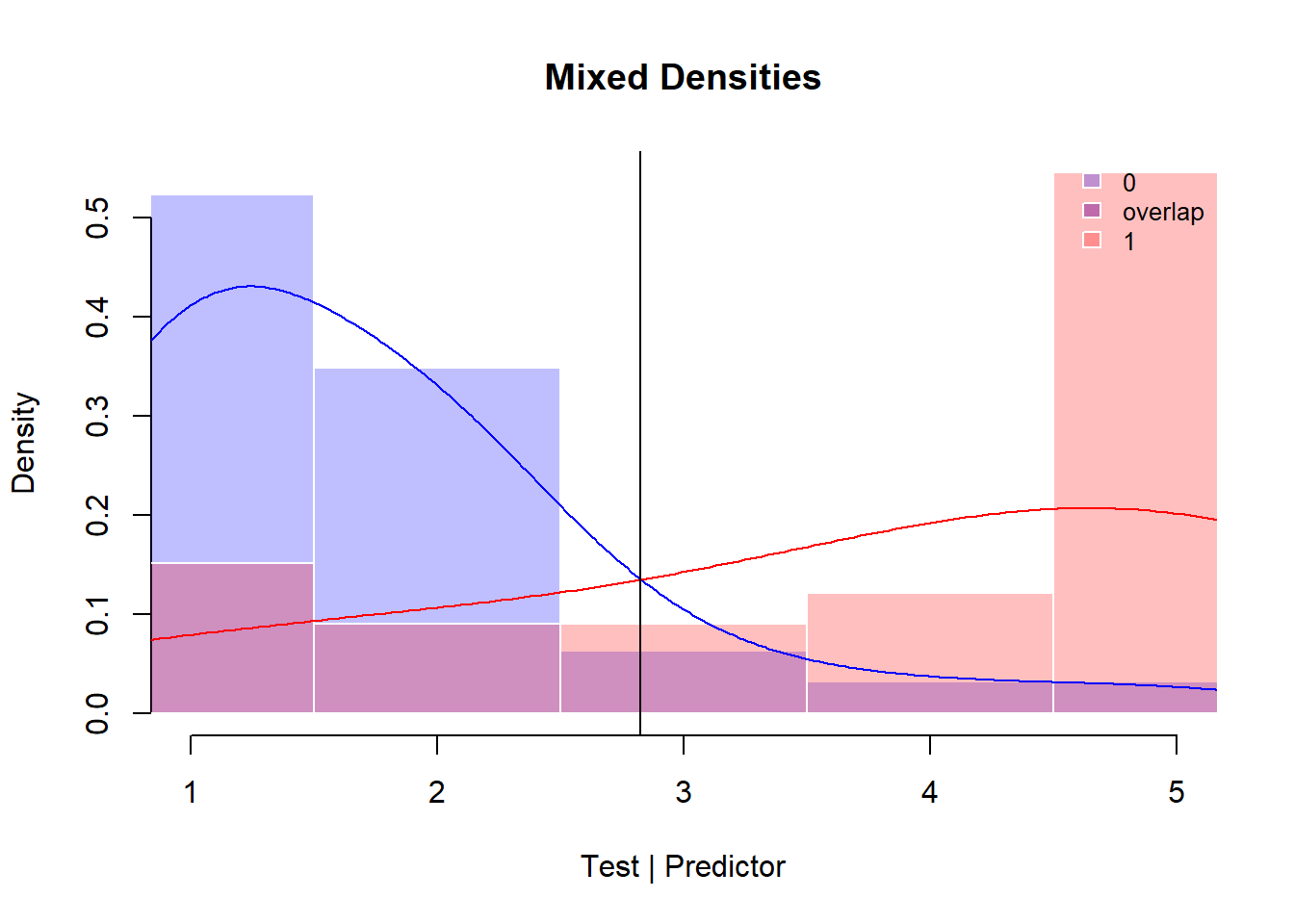Visual inspection teaches us that the score 3 is the most uncertain indicator. The radiologists used this to indicate the possibility of metastatic disease to the liver. This score is the most uncertain and is best used as an indication that further data collection is necessary. But it is also clear that all the scores provide some uncertainty concerning a decision for or against the presence of metastatic cancer.

### Using the ui.ordinal function

It should be clear that when using this few scores, all quality indices concern the limited score values and cannot be very precise. However, it is possible to calculate an interval of uncertain test scores, using a loss function. The documentation of this function provides the details. The function ui.ordinal provides additional information about possible choices for the uncertain interval:

ui.ordinal(ref=tostbegg2$d, test=tostbegg2$y, return.all=TRUE)
#> Warning in ui.ordinal(ref = tostbegg2$d, test = tostbegg2$y, return.all = TRUE): No solution found for the ui ratio constraints.
#> $Youden #> max.Youden threshold Sp Se Acc Loss C #> 0.6305916 3.0000000 0.8730159 0.7575758 0.8333333 0.3694084 0.8376623 #> #>$data.table
#>   testscores dich.thresholds d0 d1 TP FP       tpr        fpr tot TN FN
#> 1          1            >= 1 33  5 33 63 1.0000000 1.00000000  38  0  0
#> 2          2            >= 2 22  3 28 30 0.8484848 0.47619048  25 33  5
#> 3          3            >= 3  4  3 25  8 0.7575758 0.12698413   7 55  8
#> 4          4            >= 4  2  4 22  4 0.6666667 0.06349206   6 59 11
#> 5          5            >= 5  2 18 18  2 0.5454545 0.03174603  20 61 15
#>           Y
#> 1 0.0000000
#> 2 0.3722944
#> 3 0.6305916
#> 4 0.6031746
#> 5 0.5137085
#>
#> $intersection #>  3 #> #>$uncertain.interval
#>   lowerbound upperbound     UI.Sp     UI.Se    UI.Acc  UI.ratio      UI.C UI.n
#> 1          3          3 0.0000000 1.0000000 0.4285714 1.4318182 0.5000000    7
#> 2          2          3 0.8461538 0.5000000 0.7812500 0.4405594 0.6730769   32
#> 3          1          3 0.9322034 0.2727273 0.8285714 0.3559322 0.5939908   70
#> 4          3          4 0.0000000 1.0000000 0.5384615 2.2272727 0.6190476   13
#> 5          2          4 0.7857143 0.7000000 0.7631579 0.6818182 0.7607143   38
#> 6          1          4 0.9016393 0.4666667 0.8157895 0.4694486 0.6836066   76
#> 7          3          5 0.0000000 1.0000000 0.7575758 5.9659091 0.7600000   33
#> 8          2          5 0.7333333 0.8928571 0.8103448 1.7818182 0.8750000   58
#> 9          1          5 0.8730159 0.7575758 0.8333333 1.0000000 0.8376623   96
#>      MCI.Sp    MCI.Se   MCI.Acc     MCI.C MCI.n      Loss
#> 1 0.9322034 0.7333333 0.8651685 0.8375706    89 0.3333333
#> 2 0.8918919 0.8148148 0.8593750 0.8673674    64 0.5007215
#> 3 0.0000000 1.0000000 0.8461538 0.6590909    26 0.7215007
#> 4 0.9649123 0.6923077 0.8795181 0.8248988    83 0.3910534
#> 5 0.9428571 0.7826087 0.8793103 0.8627329    58 0.5584416
#> 6 0.0000000 1.0000000 0.9000000 0.5000000    20 0.7792208
#> 7 1.0000000 0.0000000 0.8730159 0.5125000    63 0.8730159
#> 8 1.0000000 0.0000000 0.8684211 0.5000000    38 1.0404040
#> 9       NaN       NaN       NaN        NA     0 1.2611833
#>
#> \$candidates
#>   lowerbound upperbound UI.Sp UI.Se    UI.Acc UI.ratio UI.C UI.n    MCI.Sp
#> 1          3          3     0     1 0.4285714 1.431818  0.5    7 0.9322034
#>      MCI.Se   MCI.Acc     MCI.C MCI.n      Loss
#> 1 0.7333333 0.8651685 0.8375706    89 0.3333333

The warning indicates that no solution is found for the given (default) ratio constraints (lower ratio .8, upper ratio 1.25). These ratio’s limit the ratio of patients with the disease and without the disease within the uncertain interval between .8 and 1.25. The selected candidate is the same as was chosen on the basis of visual inspection. The ui.ordinal function provides directly several quality indices for both possible uncertain intervals and for the test scores outside the uncertain interval (MCI’s).